##(adsbygoogle = window.adsbygoogle || []).push({}); Answer the following questions using the above information.## Let 𝑓: 𝑅 → 𝑅 be defined by 𝑓(𝑥) = 𝑥 2 is_________ (a) Neither Surjective nor Injective   (b) Surjective (c) Injective   (d) Bijective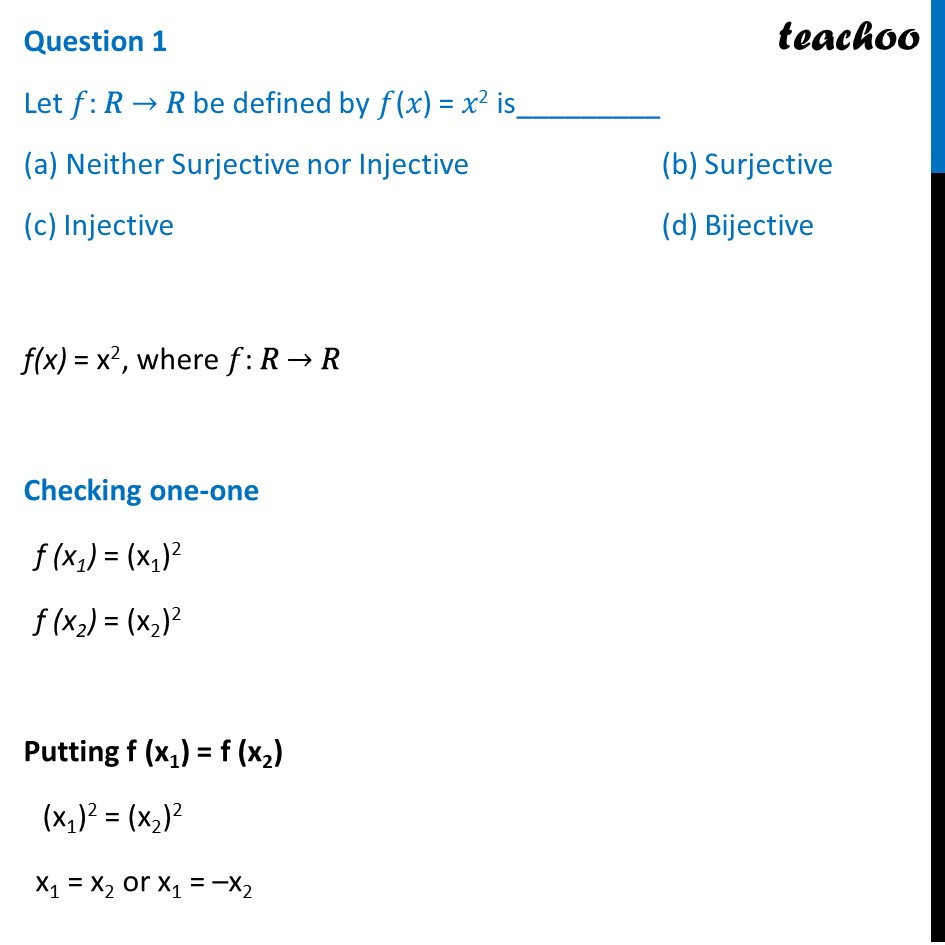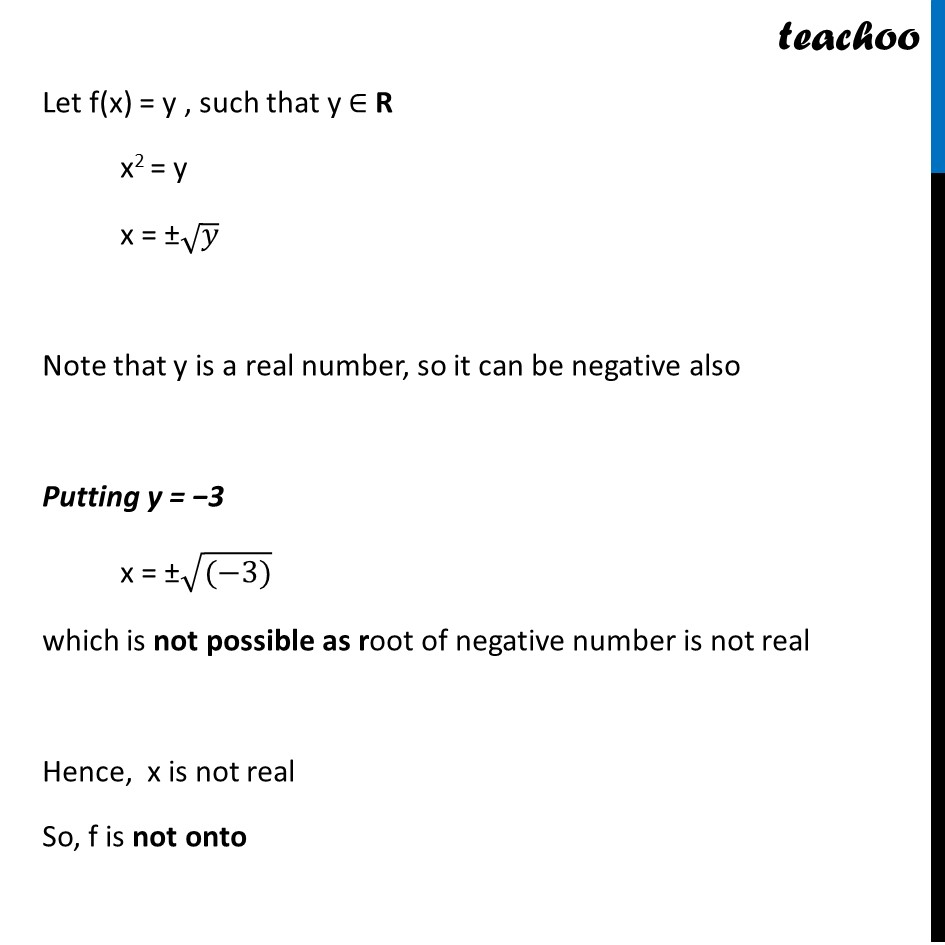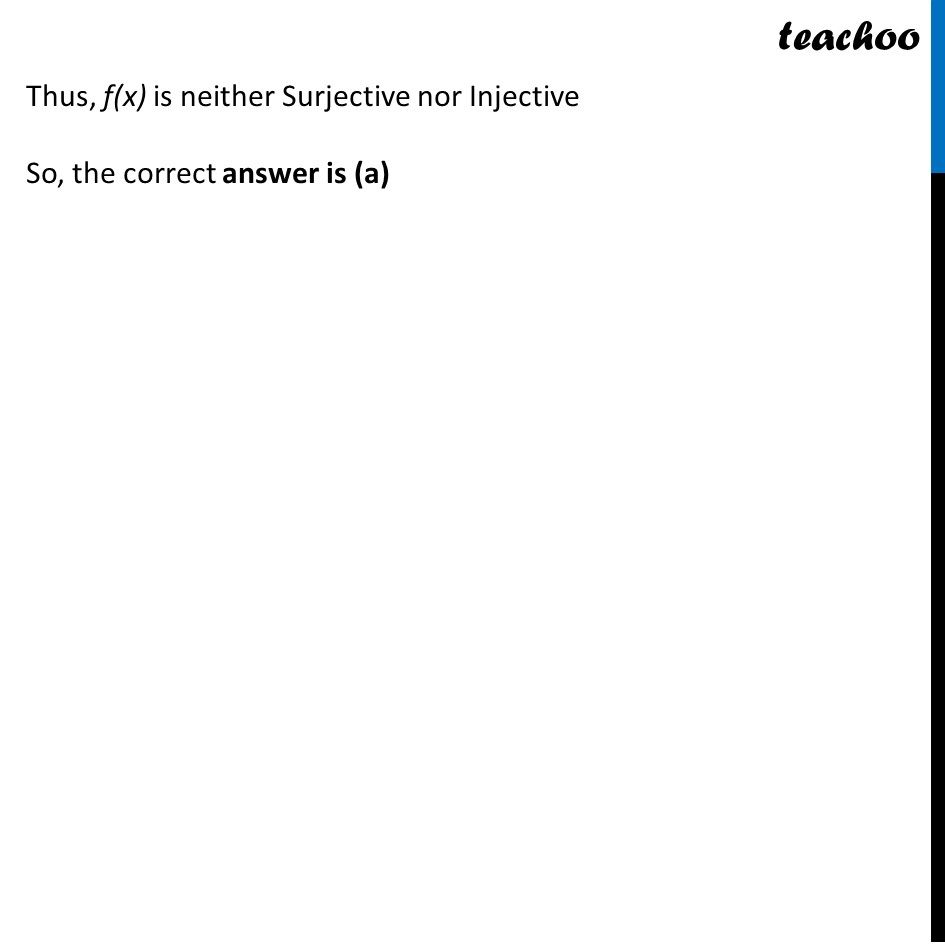## Let 𝑓: 𝑁 → 𝑁 be defined by 𝑓(𝑥) = 𝑥 2 is ________ (a) Surjective but not Injective   (b) Surjective (c) Injective   (d) Bijective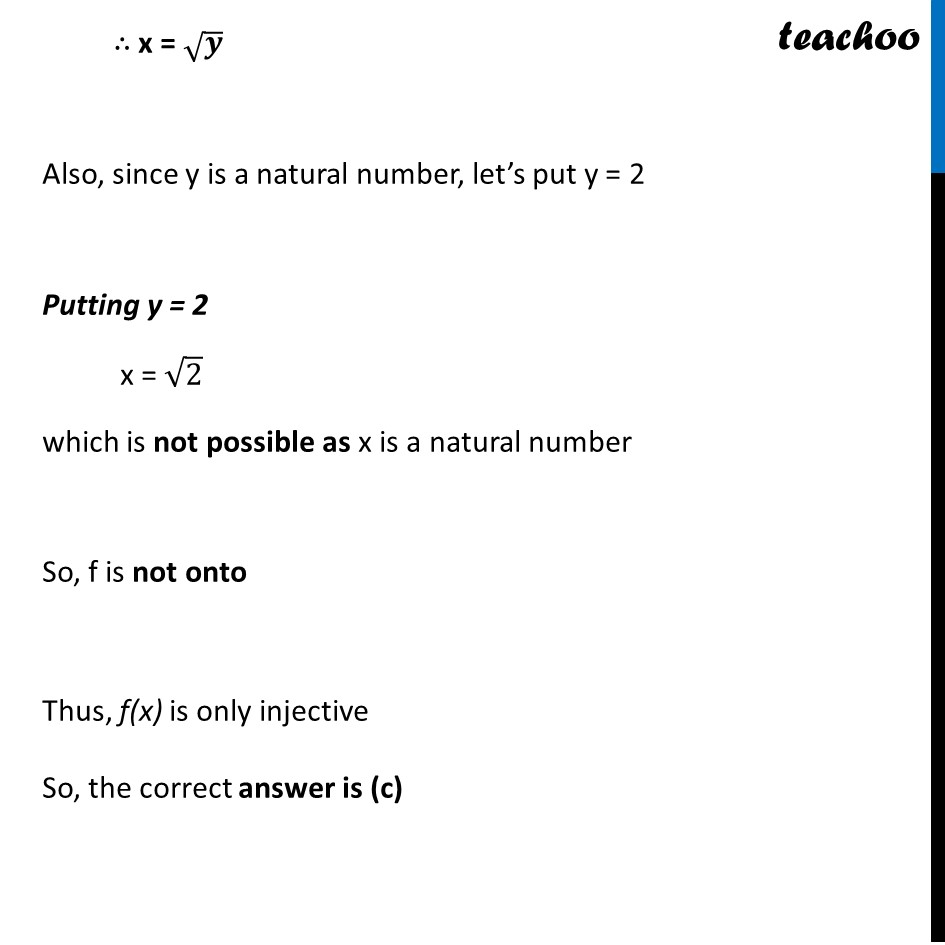## Let f: {1, 2, 3,….} → {1, 4, 9,….} be defined by 𝑓(𝑥) = 𝑥 2 is _________ (a) Bijective      (b) Surjective but not Injective (c) Injective but Surjective       (d) Neither Surjective nor Injective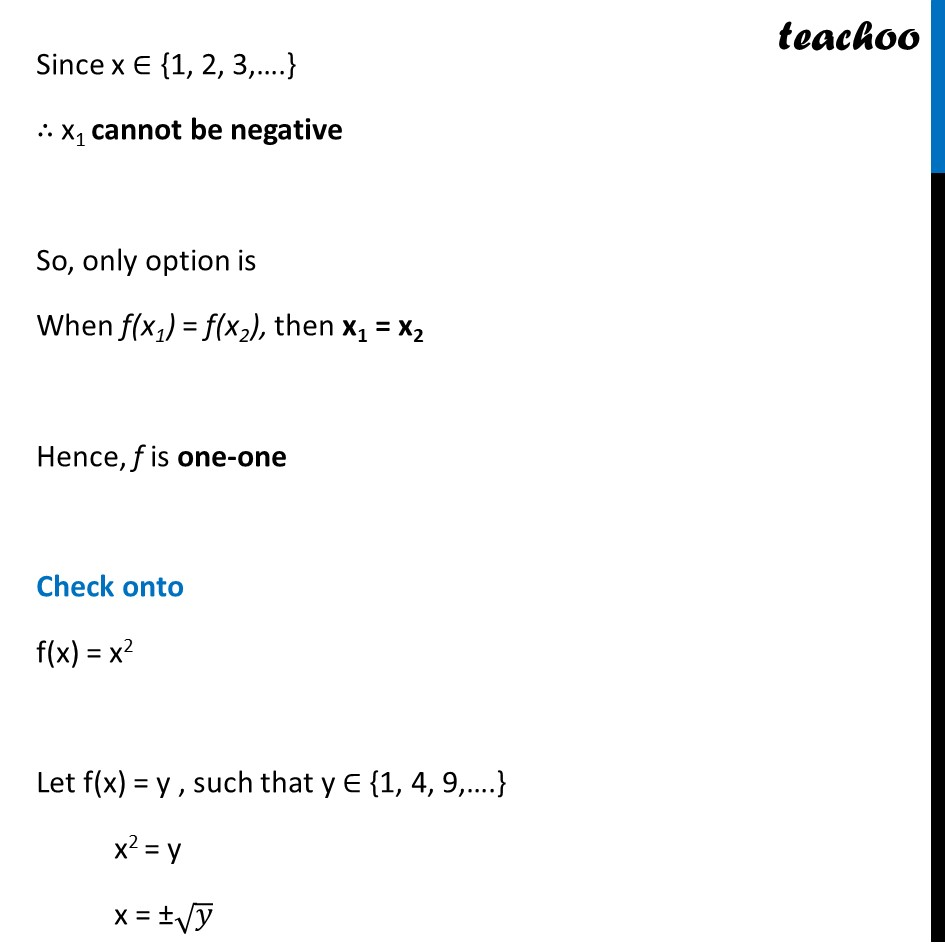## Let : 𝑁 → 𝑅 be defined by 𝑓(𝑥) = 𝑥 2 . Range of the function among the following is _________ (a) {1, 4, 9, 16,…} (b) {1, 4, 8, 9, 10,…} (c) {1, 4, 9, 15, 16,…} (d) {1, 4, 8, 16,…}## The function f: Z → Z defined by 𝑓(𝑥) = 𝑥 2 is__________ (a) Neither Injective nor Surjective    (b) Injective (c) Surjective   (d) Bijective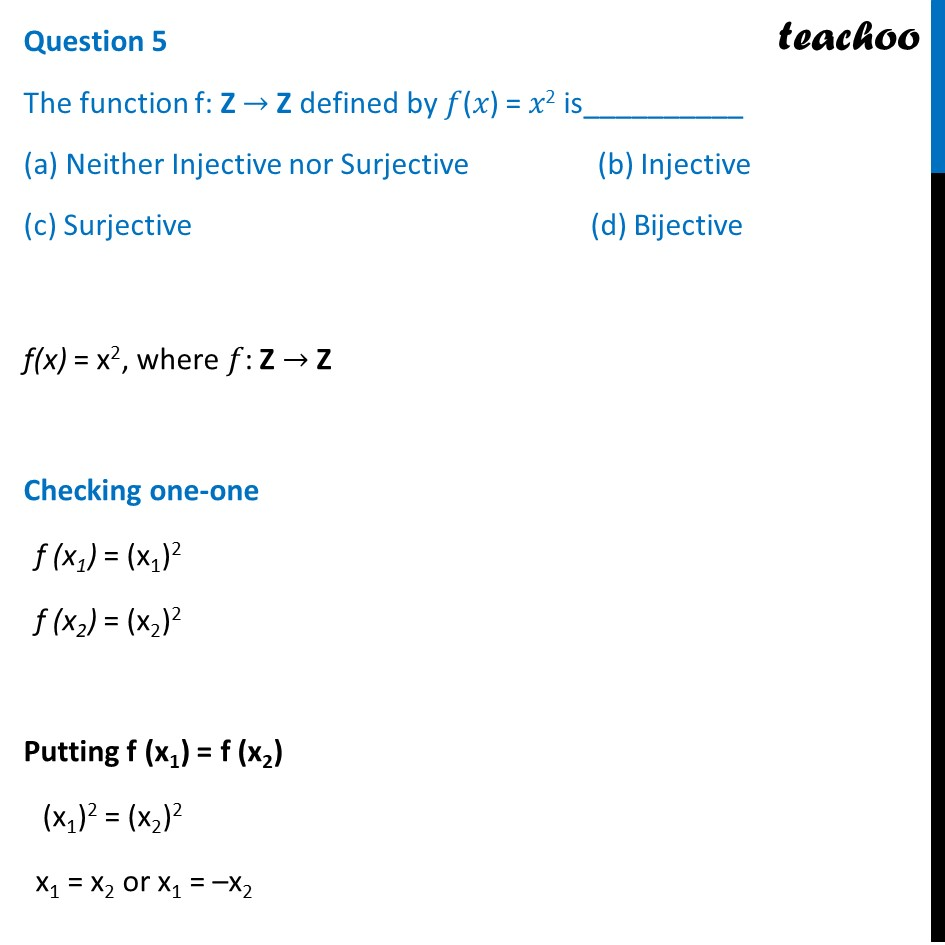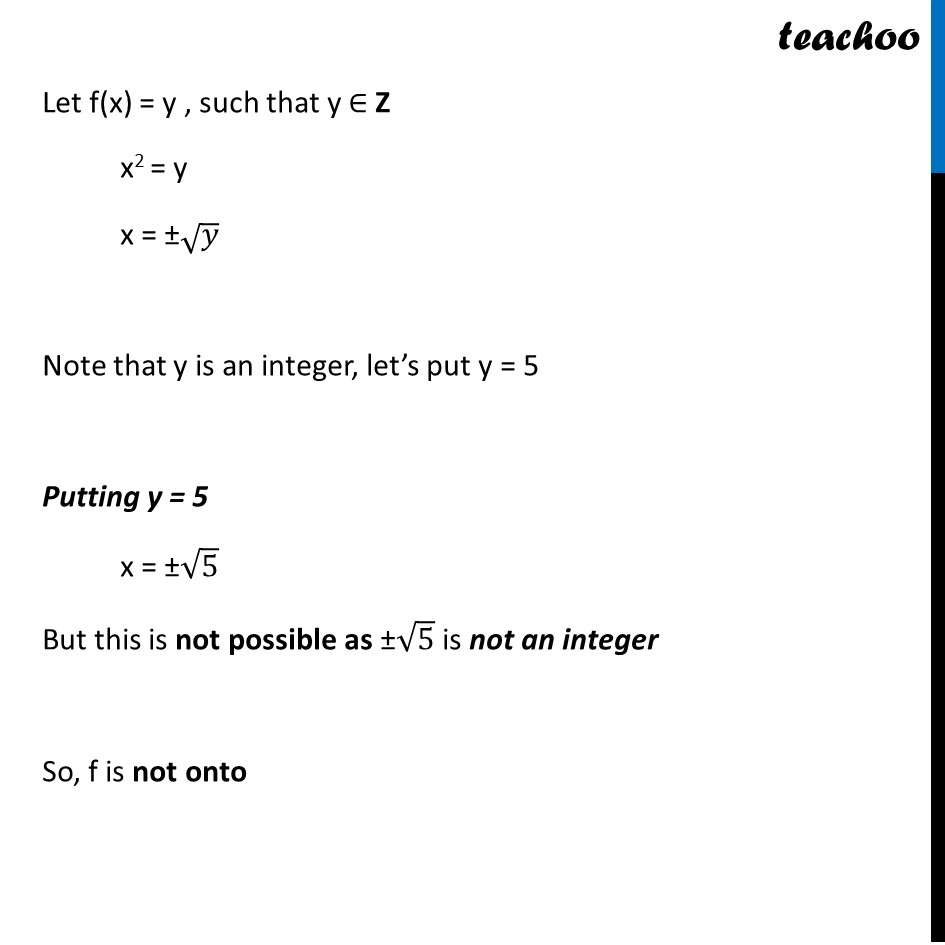1. Chapter 1 Class 12 Relation and Functions
2. Serial order wise
3. Case Based Questions (MCQ)

Transcript

Question Raji visited the Exhibition along with her family. The Exhibition had a huge swing, which attracted many children. Raji found that the swing traced the path of a Parabola as given by 𝑦 = 𝑥2 . Answer the following questions using the above information. Question 1 Let 𝑓: 𝑅 → 𝑅 be defined by 𝑓(𝑥) = 𝑥2 is_________ (a) Neither Surjective nor Injective (b) Surjective (c) Injective (d) Bijective f(x) = x2, where 𝑓: 𝑅 → 𝑅 Checking one-one f (x1) = (x1)2 f (x2) = (x2)2 Putting f (x1) = f (x2) (x1)2 = (x2)2 x1 = x2 or x1 = –x2 Since x1 does not have unique image, It is not one-one Example: f(–1) = (–1)2 = 1 f(1) = (1)2 = 1 Here, f(–1) = f(1) , but –1 ≠ 1 Hence, it is not one-one Check onto f(x) = x2 Let f(x) = y , such that y ∈ R x2 = y x = ±√𝑦 Note that y is a real number, so it can be negative also Putting y = −3 x = ±√((−3)) which is not possible as root of negative number is not real Hence, x is not real So, f is not onto Thus, f(x) is neither Surjective nor Injective So, the correct answer is (a) Question 2 Let 𝑓: 𝑁 → 𝑁 be defined by 𝑓(𝑥) = 𝑥2 is ________ (a) Surjective but not Injective (b) Surjective (c) Injective (d) Bijective f(x) = x2, where 𝑓: N → N Checking one-one f (x1) = (x1)2 f (x2) = (x2)2 Putting f (x1) = f (x2) (x1)2 = (x2)2 x1 = x2 or x1 = –x2 But x1 cannot be negative as x1 and x2 are natural numbers So, only option is When f(x1) = f(x2), then x1 = x2 Hence, f is one-one Check onto f(x) = x2 Let f(x) = y , such that y ∈ N x2 = y x = ±√𝑦 As x is natural number, it will be positive ∴ x = √𝒚 Also, since y is a natural number, let’s put y = 2 Putting y = 2 x = √2 which is not possible as x is a natural number So, f is not onto Thus, f(x) is only injective So, the correct answer is (c) Question 3 Let f: {1, 2, 3,….} → {1, 4, 9,….} be defined by 𝑓(𝑥) = 𝑥2 is _________ (a) Bijective (b) Surjective but not Injective (c) Injective but Surjective (d) Neither Surjective nor Injective f(x) = x2, where 𝑓: {1, 2, 3,….} → {1, 4, 9,….} Checking one-one f (x1) = (x1)2 f (x2) = (x2)2 Putting f (x1) = f (x2) (x1)2 = (x2)2 x1 = x2 or x1 = –x2 Since x ∈ {1, 2, 3,….} ∴ x1 cannot be negative So, only option is When f(x1) = f(x2), then x1 = x2 Hence, f is one-one Check onto f(x) = x2 Let f(x) = y , such that y ∈ {1, 4, 9,….} x2 = y x = ±√𝑦 As x ∈ {1, 2, 3,….} , it will be positive ∴ x = √𝒚 Also, since y ∈ {1, 4, 9,….} For all values of y, we will get a value of x, Where x ∈ {1, 2, 3,….} So, f is onto Thus, f(x) is one-one and onto ∴ f(x) is Bijective So, the correct answer is (a) Question 4 Let : 𝑁 → 𝑅 be defined by 𝑓(𝑥) = 𝑥2 . Range of the function among the following is _________ (a) {1, 4, 9, 16,…} (b) {1, 4, 8, 9, 10,…} (c) {1, 4, 9, 15, 16,…} (d) {1, 4, 8, 16,…} For f(x) = x2, where 𝑓: N → R Range will be = {12, 22, 32, 42, 52, 62, …} = {1, 4, 9, 16, 25, 36, …} So, the correct answer is (a) Question 5 The function f: Z → Z defined by 𝑓(𝑥) = 𝑥2 is__________ (a) Neither Injective nor Surjective (b) Injective (c) Surjective (d) Bijective f(x) = x2, where 𝑓: Z → Z Checking one-one f (x1) = (x1)2 f (x2) = (x2)2 Putting f (x1) = f (x2) (x1)2 = (x2)2 x1 = x2 or x1 = –x2 Since x1 does not have unique image, It is not one-one Example: f(–1) = (–1)2 = 1 f(1) = (1)2 = 1 Here, f(–1) = f(1) , but –1 ≠ 1 Hence, it is not one-one Check onto f(x) = x2 Let f(x) = y , such that y ∈ Z x2 = y x = ±√𝑦 Note that y is an integer, let’s put y = 5 Putting y = 5 x = ±√5 But this is not possible as ±√5 is not an integer So, f is not onto Thus, f(x) is neither Surjective nor Injective So, the correct answer is (a)

Case Based Questions (MCQ)